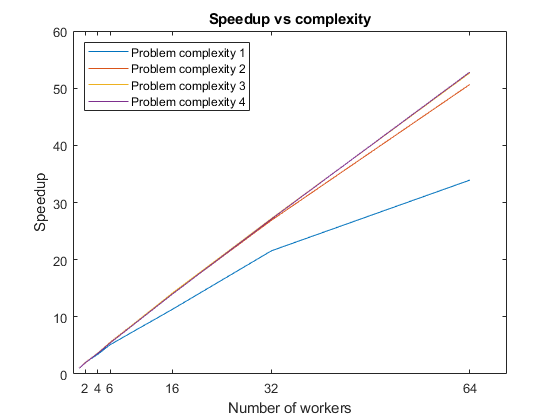# Scale Up from Desktop to Cluster

Develop your parallel MATLAB® code on your local machine and scale up to a cluster.

Clusters provide more computational resources to speed up and distribute your computations. You can run your code interactively in parallel on your local machine, then on a cluster, without changing your code. When you are done prototyping your code on your local machine, you can offload your computations to the cluster using batch jobs. So, you can close MATLAB and retrieve the results later.

Start by prototyping your algorithm on your local machine. The example uses integer factorization as a sample problem. It is a computationally intensive problem, where the complexity of the factorization increases with the magnitude of the number. You use a simple algorithm to factorize a sequence of integer numbers.

Create a vector of prime numbers in 64-bit precision, and multiply pairs of prime numbers randomly to obtain large composite numbers. Create an array to store the results of each factorization. The code in each of the following sections in this example can take more than 20 min. To make it faster, reduce the workload by using fewer prime numbers, such as `2^19`. Run with `2^21` to see the optimum final plots.

```primeNumbers = primes(uint64(2^21)); compositeNumbers = primeNumbers.*primeNumbers(randperm(numel(primeNumbers))); factors = zeros(numel(primeNumbers),2);```

Use a loop to factor each composite number, and measure the time that the computation takes.

```tic; for idx = 1:numel(compositeNumbers) factors(idx,:) = factor(compositeNumbers(idx)); end toc```
```Elapsed time is 684.464556 seconds. ```

### Run Your Code on a Local Parallel Pool

Parallel Computing Toolbox™ enables you to scale up your workflow by running on multiple workers in a parallel pool. The iterations in the previous `for` loop are independent, and so you can use a `parfor` loop to distribute iterations to multiple workers. Simply transform your `for` loop into a `parfor` loop. Then, run the code and measure the overall computation time. The code runs in a parallel pool with no further changes, and the workers send your computations back to the local workspace. Because the workload is distributed across several workers, the computation time is lower.

```tic; parfor idx = 1:numel(compositeNumbers) factors(idx,:) = factor(compositeNumbers(idx)); end toc```
```Elapsed time is 144.550358 seconds. ```

When you use `parfor` and you have Parallel Computing Toolbox, MATLAB automatically starts a parallel pool of workers. The parallel pool takes some time to start. This example shows a second run with the pool already started.

The default profile is `'Processes'`. You can check that this profile is set as default on the MATLAB Home tab, in Parallel > Select Parallel Environment. With this profile enabled, MATLAB creates workers on your machine for the parallel pool. When you use the `'Processes'` profile, MATLAB, by default, starts as many workers as physical cores in your machine, up to your preferred number of workers. You can control parallel behavior using the parallel preferences. On the MATLAB Home tab, select Parallel > Parallel Preferences.

To measure the speedup with the number of workers, run the same code several times, limiting the maximum number of workers. First, define the number of workers for each run, up to the number of workers in the pool, and create an array to store the result of each test.

```numWorkers = [1 2 4 6]; tLocal = zeros(size(numWorkers));```

Use a loop to iterate through the maximum number of workers, and run the previous code. To limit the number of workers, use the second input argument of `parfor`.

```for w = 1:numel(numWorkers) tic; parfor (idx = 1:numel(compositeNumbers), numWorkers(w)) factors(idx,:) = factor(compositeNumbers(idx)); end tLocal(w) = toc; end```

Calculate the speedup by computing the ratio between the computation time of a single worker and the computation time of each maximum number of workers. To visualize how the computations scale up with the number of workers, plot the speedup against the number of workers. Observe that the speedup increases with the number of workers. However, the scaling is not perfect due to overhead associated with parallelization.

```f = figure; speedup = tLocal(1)./tLocal; plot(numWorkers, speedup); title('Speedup with the number of workers'); xlabel('Number of workers'); xticks(numWorkers); ylabel('Speedup');```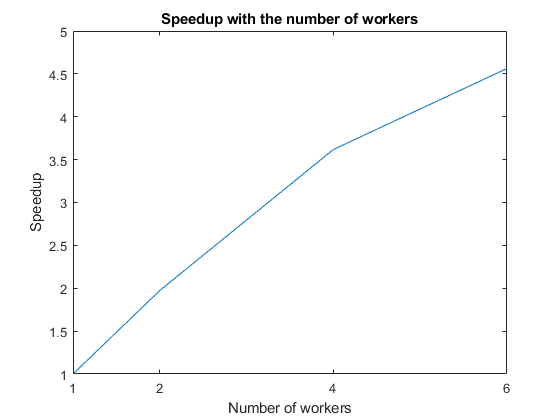When you are done with your computation, delete the current parallel pool so you can create a new one for your cluster. You can obtain the current parallel pool with the `gcp` function.

`delete(gcp);`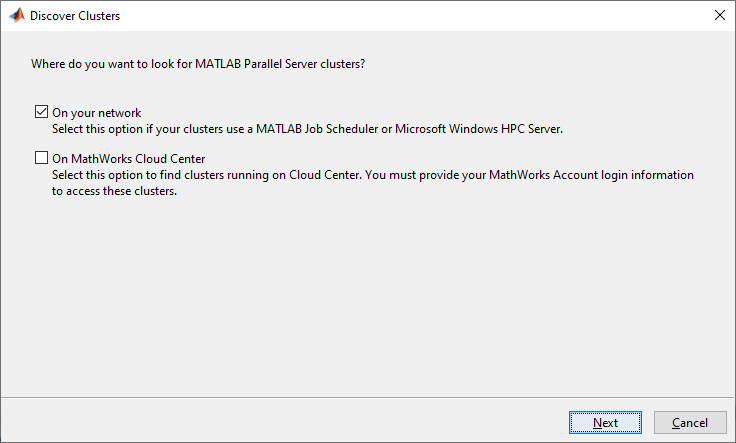After you set up a cluster profile, you can modify its properties in Parallel > Create and Manage Clusters. For more information, see Discover Clusters and Use Cluster Profiles. The following image shows a cluster profile in the Cluster Profile Manager: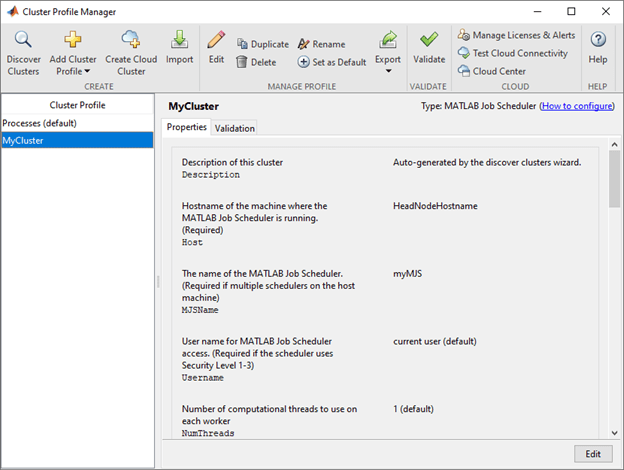### Run Your Code on a Cluster Parallel Pool

If you want to run parallel functions in the cluster by default, set your cluster profile as default in Parallel > Select Parallel Environment: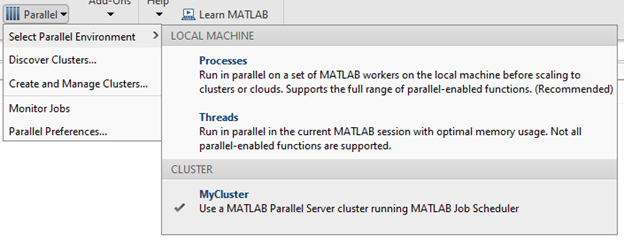You can also use a programmatic approach to specify your cluster. To do so, start a parallel pool in the cluster by specifying the name of your cluster profile in the `parpool` command. In the following code, replace `MyCluster` with the name of your cluster profile. Also specify the number of workers with the second input argument.

`parpool('MyCluster',64);`
```Starting parallel pool (parpool) using the 'MyCluster' profile ... connected to 64 workers. ```

As before, measure the speedup with the number of workers by running the same code several times, and limiting the maximum number of workers. Because the cluster in this example allows for more workers than the local setup, `numWorkers` can hold more values. If you run this code, the `parfor` loop now runs in the cluster.

```numWorkers = [1 2 4 6 16 32 64]; tCluster = zeros(size(numWorkers)); for w = 1:numel(numWorkers) tic; parfor (idx = 1:numel(compositeNumbers), numWorkers(w)) factors(idx,:) = factor(compositeNumbers(idx)); end tCluster(w) = toc; end```

Calculate the speedup, and plot it against the number of workers to visualize how the computations scale up with the number of workers. Compare the results with those of the local setup. Observe that the speedup increases with the number of workers. However, the scaling is not perfect due to overhead associated with parallelization.

```figure(f); hold on speedup = tCluster(1)./tCluster; plot(numWorkers, speedup); title('Speedup with the number of workers'); xlabel('Number of workers'); xticks(numWorkers(2:end)); ylabel('Speedup');```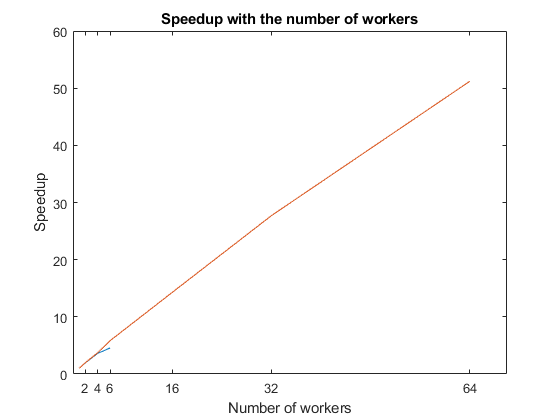When you are done with your computations, delete the current parallel pool.

`delete(gcp);`

### Offload and Scale Your Computations with `batch`

After you are done prototyping and running interactively, you can use batch jobs to offload the execution of long-running computations in the background with batch processing. The computation happens in the cluster, and you can close MATLAB and retrieve the results later.

Use the `batch` function to submit a batch job to your cluster. You can place the contents of your algorithm in a script, and use the `batch` function to submit it. For example, the script myParallelAlgorithm performs a simple benchmark based on the integer factorization problem shown in this example. The script measures the computation time of several problem complexities with different number of workers.

Note that if you send a script file using `batch`, MATLAB transfers all the workspace variables to the cluster, even if your script does not use them. If you have a large workspace, it impacts negatively the data transfer time. As a best practice, convert your script to a function file to avoid this communication overhead. You can do this by simply adding a function line at the beginning of your script. To learn how to convert myParallelAlgorithm to a function, see myParallelAlgorithmFcn.

The following code submits `myParallelAlgorithmFcn `as a batch job. `myParallelAlgorithmFcn` returns two output arguments, `numWorkers` and `time`, and you must specify `2` as the number of outputs input argument. Because the code needs a parallel pool for the `parfor` loop, use the `'Pool'` name-value pair in `batch` to specify the number of workers. The cluster uses an additional worker to run the function itself. By default, `batch `changes the current folder of the workers in the cluster to the current folder of the MATLAB client. It can be useful to control the current folder. For example, if your cluster uses a different filesystem, and therefore the paths are different, such as when you submit from a Windows client machine to a Linux cluster. Set the name-value pair `'CurrentFolder' `to a folder of your choice, or to `'.'` to avoid changing the folder of the workers.

```totalNumberOfWorkers = 65; cluster = parcluster('MyCluster'); job = batch(cluster,'myParallelAlgorithmFcn',2,'Pool',totalNumberOfWorkers-1,'CurrentFolder','.');```

To monitor the state of your job after it is submitted, open the Job Monitor in Parallel > Monitor Jobs. When computations start in the cluster, the state of the job changes to `running`: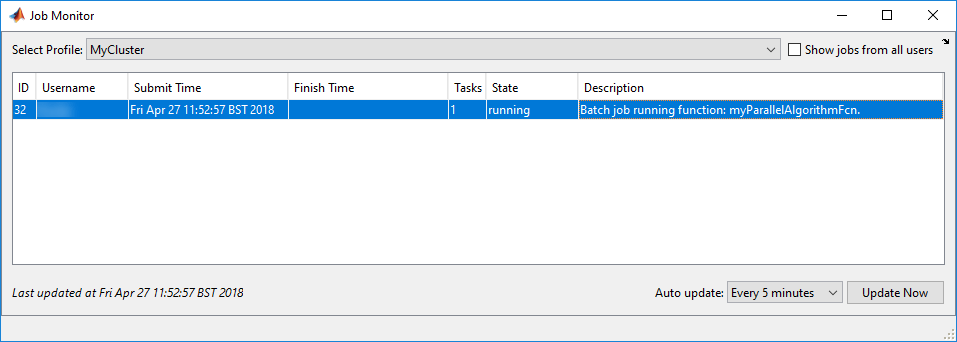You can close MATLAB after the job has been submitted. When you open MATLAB again, the Job Monitor keeps track of the job for you, and you can interact with it if you right-click it. For example, to retrieve the job object, select Show Details, and to transfer the outputs of the batch job into the workspace, select Fetch Outputs.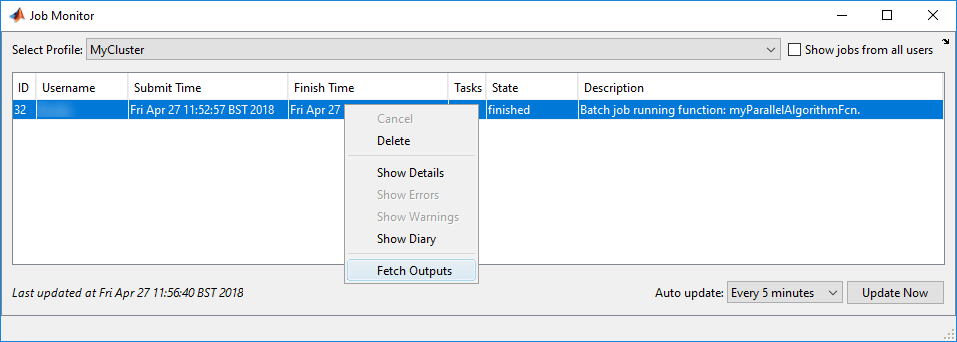Alternatively, if you want to block MATLAB until the job completes, use the `wait` function on the job object.

`wait(job);`

To transfer the outputs of the function from the cluster, use the `fetchOutputs` function.

```outputs = fetchOutputs(job); numWorkers = outputs{1}; time = outputs{2};```

After retrieving the results, you can use them for calculations on your local machine. Calculate the speedup, and plot it against the number of workers. Because the code runs factorizations for different problem complexities, you get a plot for each level. You can see that, for each problem complexity, the speedup increases with the number of workers, until the overhead for additional workers is greater than the performance gain from parallelization. As you increase the problem complexity, you achieve better speedup at large numbers of workers, because overhead associated with parallelization is less significant.

```figure speedup = time(1,:)./time; plot(numWorkers,speedup); legend('Problem complexity 1','Problem complexity 2','Problem complexity 3','Problem complexity 4','Location','northwest'); title('Speedup vs complexity'); xlabel('Number of workers'); xticks(numWorkers(2:end)); ylabel('Speedup');```